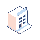• 料金

# how to set if condiction for dictionry value in qweb

i have a loop on dict doc

`<t t-if="doc[currency_id] != 'SDG'">  <t t-set="total1" t-value="total1+ doc['tuition']"/></t>`

2 コメント<t t-set="total1" t-value="0"/>

<t t-if="doc.currency_id.display_name != 'SDG'">

<t t-set="total1" t-value="total1+ doc['tuition']"/>

</t>

##### 2 回答

Try this :
<t t-if="doc['currency_id'] !== 'SDG'">
<t t-set="total1" t-value="total1+ doc['tuition']"/>
</t>

This might work.You can do it as like you are doing in python.

`<t t-if="doc['currency_id'] != 'SDG'">  <t t-set="total1" t-value="total1+ doc['tuition']"/></t>`
2 コメントit does not work , i get

Error to render compiling AST

KeyError: None

Template: 754

Path: /templates/t/t/div/table/tbody/t/tr/td/t

Node: <t t-if="doc[currency_id] != 'SDG'">

<t t-set="total1" t-value="total1+ doc['tuition']"/>

</t>